# Sharpe Ratio

## A Sharper Sharpe: Its Biased.

In a series of posts, we looked at Damien Challet's 'Sharper estimator' of the Signal-Noise Ratio, which computes the number of drawdowns in the returns series (plus some permutations thereof), then uses a spline function to infer the Signal-Noise Ratio from the drawdown statistic. The spline function is built from the results of Monte Carlo simulations.

In the last post of the series, we looked at the apparent bias of this 'drawdown estimator'. We suggested, somewhat facetiously, that one could achieve similar properties to the drawdown estimator (reduced RMSE, bias, etc.) by taking the traditional moment-based Sharpe ratio and multiplying it by 0.8.

I contacted Challet to present my concerns. I suspected that the spline function was trained with too narrow a range of population Signal-Noise ratios, which would result in this bias, and suggested he expand his simulations as a fix. I see that since that time the sharpeRratio package has gained a proper github page, and the version number was bumped to 1.2. (It has not been released to CRAN, so it is premature to call it "the" 1.2 release.)

In this post, I hope to:

1. Demonstrate the bias of the drawdown estimator in a way that clearly illustrates why "Sharpe ratio times 0.8" (well, really 0.7) is a valid comparison.
2. Check whether the bias has been corrected in the 1.2 development version. (Spoiler alert: no.)
3. Provide further evidence that the issue is the spline function, and not in the estimation of $$\nu$$.

In order to compare two versions of the same package in the same R session, I forked the github repo, and made a branch with a renamed package. I have called it sharpeRratioTwo because I do not expect it to be used by anyone, and because naming is still a hard problem in CS. To install the package to play along, one can:

library(devtools)
devtools::install_github('shabbychef/sharpeRratio',ref='astwo')


First, I perform some simulations. I draw 128 days of daily returns from a $$t$$ distribution with $$\nu=4$$ degrees of freedom. I then compute: the moment-based Sharpe ratio; the moment-based Sharpe ratio, but debiased using higher order moments; the drawdown estimator from the 1.1 version of the package, as installed from CRAN; the drawdown estimator from the 1.2 version of the package; the drawdown estimator from the 1.2 version of the package, but feeding $$\nu$$ to the estimator. I do this for 20000 draws of returns. I repeat for 256, and 512 days of data, and for the population Signal-Noise ratio varying from 0.25 to 1.5 in "annualized units" (per square root year), assuming 252 trading days per year. I use doFuture to run the simulations in parallel.

suppressMessages({
library(dplyr)
library(tidyr)
library(tibble)
library(SharpeR)
library(sharpeRratio)
library(sharpeRratioTwo)
# https://cran.r-project.org/web/packages/doFuture/vignettes/doFuture.html
library(doFuture)
registerDoFuture()
plan(multiprocess)
})
# only works for scalar pzeta:
onesim <- function(nday,pzeta=0.1,nu=4) {
x <- pzeta + sqrt(1 - (2/nu)) * rt(nday,df=nu)
srv <- SharpeR::as.sr(x,higher_order=TRUE)
# mental note: this is much more awkward than it should be,
# let's make it easier in SharpeR!
ssr <- srv$sr ssr_b <- ssr - SharpeR::sr_bias(snr=ssr,n=nday,cumulants=srv$cumulants)

ssr <- mean(x) / sd(x)
sim <- sharpeRratio::estimateSNR(x)
twm <- sharpeRratioTwo::estimateSNR(x)
# this cheats and gives the true nu to the estimator
cht <- sharpeRratioTwo::estimateSNR(x,nu=nu)
c(ssr,ssr_b,sim$SNR,twm$SNR,cht$SNR) } repsim <- function(nrep,nday,pzeta=0.1,nu=4) { dummy <- invisible(capture.output(jumble <- replicate(nrep,onesim(nday=nday,pzeta=pzeta,nu=nu)),file='/dev/null')) retv <- t(jumble) colnames(retv) <- c('sr','sr_unbiased','ddown','ddown_two','ddown_cheat') invisible(as.data.frame(retv)) } manysim <- function(nrep,nday,pzeta,nu=4,nnodes=5) { if (nrep > 2*nnodes) { # do in parallel. nper <- table(1 + ((0:(nrep-1) %% nnodes))) retv <- foreach(i=1:nnodes,.export = c('nday','pzeta','nu')) %dopar% { repsim(nrep=nper[i],nday=nday,pzeta=pzeta,nu=nu) } %>% bind_rows() } else { retv <- repsim(nrep=nrep,nday=nday,pzeta=pzeta,nu=nu) } retv } # summarizing function sim_summary <- function(retv) { retv %>% tidyr::gather(key=metric,value=value,-pzeta,-nday) %>% filter(!is.na(value)) %>% group_by(pzeta,nday,metric) %>% summarize(meanvalue=mean(value), serr=sd(value) / sqrt(n()), rmse=sqrt(mean((pzeta - value)^2)), nsims=n()) %>% ungroup() %>% arrange(pzeta,nday,metric) } ope <- 252 pzeta <- seq(0.25,1.5,by=0.25) / sqrt(ope) params <- tidyr::crossing(tibble::tribble(~nday,128,256,512), tibble::tibble(pzeta=pzeta)) nrep <- 20000 # can do 2000 in ~20 minutes using 7 nodes. set.seed(1234) system.time({ results <- params %>% group_by(nday,pzeta) %>% summarize(sims=list(manysim(nrep=nrep,nnodes=7,pzeta=pzeta,nday=nday))) %>% ungroup() %>% tidyr::unnest() })   user system elapsed 79879.368 20.066 28291.224  (Don't trust those timings, it should only take 3 and a half hours on 7 cores, but I hibernated my laptop in the middle.) I compute the mean of each estimator over the 20000 draws, divide that mean estimate by the true Signal-Noise Ratio, then plot versus the annualized SNR. I plot errobars at plus and minus one standard error around the mean. I believe this plot is more informative than previous versions, as it clearly shows the geometric bias of the drawdown estimator. As promised, we see that the drawdown estimator consistently estimates a value around 70% of the true value. This geometric bias appears constant across the range of SNR values we tested. Moreover, it is apparently not affected by sample size: we see about the same bias for 2 years of data as we do for half a year of data. The moment estimator, on the other hand, shows a slight positive bias which is decreasing in sample size, as described by Bao and Miller and Gehr. The higher order moment correction mitigates this bias somewhat, but does not appear to eliminate it entirely. We also see that the bias of the drawdown estimator is not fixed in the most recent version of the package, and does not appear to due to estimation of the $$\nu$$ parameter. On the contrary, the estimation of $$\nu$$ appears to make the bias worse. We conclude that the drawdown estimator is still biased, and we suggest that practicioners do not use this estimator until this issue is resolved. ph <- results %>% sim_summary() %>% mutate(metric=case_when(.$metric=='ddown' ~ 'drawdown estimator v1.1',
.$metric=='ddown_two' ~ 'drawdown estimator v1.2', .$metric=='ddown_cheat' ~ 'drawdown estimator v1.2, nu given',
.$metric=='sr_unbiased' ~ 'moment estimator, debiased', .$metric=='sr' ~ 'moment estimator (SR)',
TRUE ~ 'error')) %>%
mutate(bias = meanvalue / pzeta,
zeta_pa=sqrt(ope) * pzeta,
serr = serr / pzeta) %>%
ggplot(aes(zeta_pa,bias,color=metric,ymin=bias-serr,ymax=bias+serr)) +
geom_line() + geom_point() + geom_errorbar(alpha=0.5) +
geom_hline(yintercept=1,linetype=2,alpha=0.5) +
facet_wrap(~nday,labeller=label_both) +
scale_y_log10() +
labs(x='Signal-noise ratio (per square root year)',
y='empirical expected value of estimator, divided by actual value',
color='estimator',
title='geometric bias of SR estimators')
print(ph)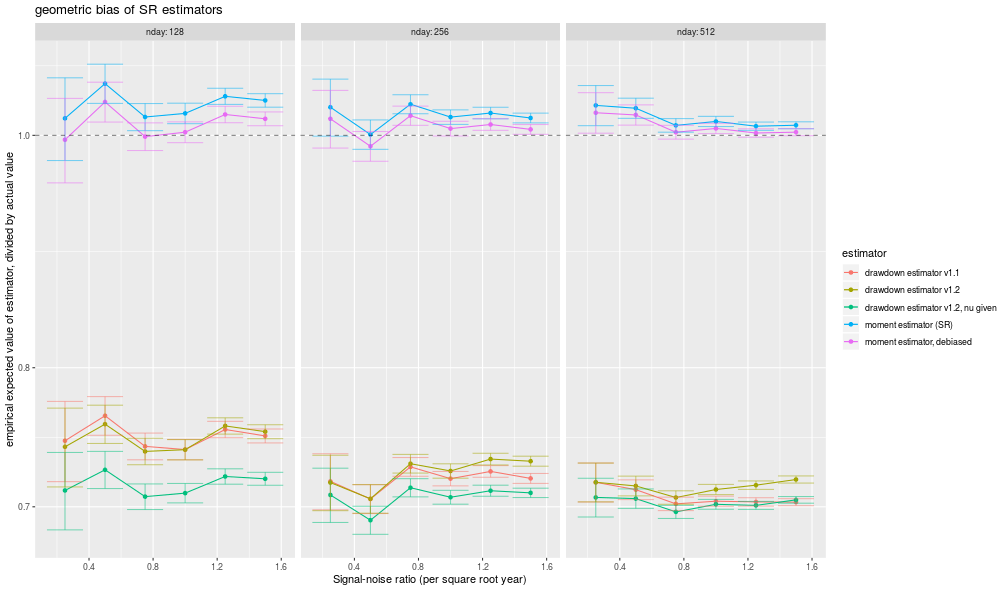## A Sharper Sharpe: Just Shrink it!

In a series of blog posts we have looked at Damien Challet's 'Sharper estimator' of the Signal-Noise Ratio, finding it to have lower mean square error than the Sharpe ratio (which we are also calling the 'moment based estimator') for symmetric heavy tailed and skewed returns distributions; in a later post, we compared it to two other estimators for the case of $$t$$-distributed returns. In that last post we noticed that for a substantially easier problem (estimating the mean of a shifted, rescaled $$t$$ when the degrees of freedom and rescaling factor are known), the drawdown estimator seems to achieve lower mean square error than the Cramér Rao lower bound.

From this puzzling result, I suspected that the drawdown estimator is performing some kind of subconcious shrinkage. That is, the drawdown estimator estimates Signal Noise ratio by computing the average, under multiple permutations, number of drawdown and drawup records, then feeds these into a spline function which was built on simulations to map back to Signal Noise ratio. The problem is this reverse mapping may have been built on a limited parameter set with, say, a least squares fit. Values outside of the training range will be pulled back into the training range.

To be concrete, if the training simulations only included Signal Noise ratios between $$-1.5$$ and $$1.5$$ annualized, say, and the process were tested on returns with a Signal Noise ratio of $$3.5$$, say (these are comically large values for illustration purposes), then the spline function would very likely suggest the value is $$1.5$$. While this seems like a catastrope in our hypothetical situation, if you happen to test the estimator on populations which are within the range of Signal Noise ratios used in calibration, you are likely to see reduced mean square error, as we have in our simulations.

Rather than advocate for or against this particular choice of bias-variance tradeoff, here we consider an alternative shrinkage estimator: we take the vanilla Sharpe ratio and multiply it by 0.80. Here we test this basic shrinkage estimator against the Sharpe ratio and the drawdown estimator over a range of sample sizes, Signal Noise ratios, and kurtosis factor for returns drawn from normal or $$t$$ distributions, as in our first study. We compute the empirical root mean square error of each estimator along with the empirical bias over 500 simulations for each parameter setting.

suppressMessages({
library(dplyr)
library(tidyr)
library(tibble)
library(sharpeRratio)
# https://cran.r-project.org/web/packages/doFuture/vignettes/doFuture.html
library(doFuture)
registerDoFuture()
plan(multiprocess)
})

onesim <- function(n,pzeta,gen=rnorm,...) {
x <- gen(n) + pzeta
mux <- mean(x)
sdx <- sd(x)
mome <- (mux + (pzeta - pzeta)) / sdx
sim$SNR })) cbind(pzeta,mome,ddsr) } repsim <- function(nrep,n,pzeta,gen=rnorm) { dummy <- invisible(capture.output(jumble <- replicate(nrep,onesim(n=n,pzeta=pzeta,gen=gen)),file='/dev/null')) retv <- aperm(jumble,c(1,3,2)) dim(retv) <- c(nrep * length(pzeta),dim(jumble)) colnames(retv) <- colnames(jumble) invisible(as.data.frame(retv)) } manysim <- function(nrep,n,pzeta,ex.kurt=0,nnodes=5) { stopifnot(ex.kurt >= 0) # not yet if (ex.kurt==0) { gen <- rnorm } else { thedf <- 4 + 6 / ex.kurt rescal <- sqrt((thedf - 2)/thedf) gen <- function(n) { rescal * rt(n,df=thedf) } } if (nrep > 2*nnodes) { # do in parallel. nper <- table(1 + ((0:(nrep-1) %% nnodes))) retv <- foreach(i=1:nnodes,.export = c('n','pzeta','gen','onesim','repsim')) %dopar% { repsim(nrep=nper[i],n=n,pzeta=pzeta,gen=gen) } %>% bind_rows() } else { retv <- repsim(nrep=nrep,n=n,pzeta=pzeta,gen=gen) } retv } ope <- 252 pzetasq <- c(0,1/4,1,4) / ope pzeta <- sqrt(pzetasq) params <- tidyr::crossing(tibble::tribble(~n,100,200,400,800,1600), tibble::tribble(~kurty,0,4,16,64), tibble::tibble(pzeta=pzeta)) # run it nrep <- 500 set.seed(1234) system.time({ results <- params %>% group_by(n,kurty,pzeta) %>% summarize(sims=list(manysim(nrep=nrep,nnodes=6, pzeta=pzeta,n=n,ex.kurt=kurty))) %>% ungroup() %>% tidyr::unnest() })   user system elapsed 12503.75 4537.97 2532.52  Now I collect the results of the simulation, computing the bias, standard error and root mean square error of the two methods of computing Sharpe. # summarize the moments shrinkage_amt <- 0.80 sumres <- results %>% mutate(shrn=shrinkage_amt * mome) %>% # "shrinkage" group_by(pzeta,n,kurty) %>% summarize(bias_mome=mean(mome - pzeta,na.rm=TRUE), rmse_mome=sqrt(mean((mome - pzeta)^2,na.rm=TRUE)), bias_shrn=mean(shrn - pzeta,na.rm=TRUE), rmse_shrn=sqrt(mean((shrn - pzeta)^2,na.rm=TRUE)), bias_ddsr=mean(ddsr - pzeta,na.rm=TRUE), rmse_ddsr=sqrt(mean((ddsr - pzeta)^2,na.rm=TRUE))) %>% ungroup() %>% arrange(pzeta,n,kurty) %>% tidyr::gather(key=series,value=value,matches('_(mome|ddsr|shrn)$')) %>%
tidyr::separate(series,into=c('metric','stat')) %>%
mutate(stat=case_when(.$stat=='mome' ~ 'moment based estimator', .$stat=='ddsr' ~ 'drawdown based estimator',
.$stat=='shrn' ~ 'shrunk moment based estimator', TRUE ~ 'bad code')) %>% mutate(annualized SNR=signif(pzeta * sqrt(ope),digits=2)) %>% rename(excess kurtosis=kurty)  Here we plot the RMSE versus sample size. The new shrinkage moment based estimator, plotted in blue, appears to achieve about the same mean square error than the drawdown based estimator, sometimes a little lower, sometimes a little higher. # plot library(ggplot2) ph <- sumres %>% filter(metric=='rmse') %>% mutate(value=sqrt(ope) * value) %>% # annualized ggplot(aes(n,value,color=stat)) + geom_line() + geom_point() + scale_x_log10() + scale_y_log10() + facet_grid(annualized SNR~excess kurtosis,labeller=label_both) + labs(y='RMSE of estimator of SNR, annualized', x='number of days of data', title='empirical root mean squared errors of three ways of estimating Signal Noise ratio') print(ph)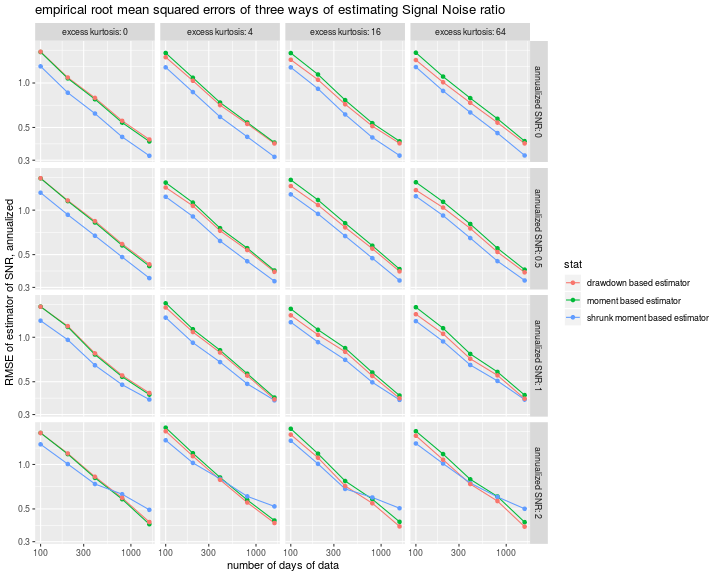Here we plot the bias (in annualized units) of the three estimators. The traditional Sharpe ratio is the only estimator among the three that appears to be nearly unbiased. The drawdown estimator is nearly unbiased for the case of normal returns, but has about the same sizable bias for $$t$$ returns. # plot library(ggplot2) ph <- sumres %>% filter(metric=='bias') %>% mutate(value=sqrt(ope) * value) %>% # annualized ggplot(aes(n,value,color=stat)) + geom_line() + geom_point() + scale_x_log10() + facet_grid(annualized SNR~excess kurtosis,labeller=label_both) + labs(y='bias of estimator of SNR, annualized', x='number of days of data', title='empirical bias of three ways of estimating Signal Noise ratio') print(ph)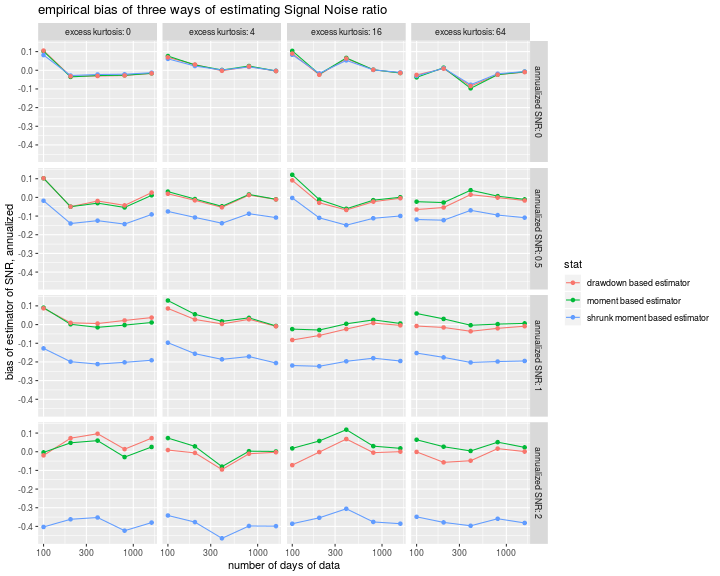We are not suggesting that quant managers take a 20% haircut off of their Sharpe ratios! (Although perhaps that's reasonable for backtests.) The point is that a very simple, and truly repugnant, modification to the Sharpe ratio can achieve the same empirical performance as the drawdown estimator. At the same time, the latter lacks any theoretical justification for improved efficiency. Together these cast serious doubts on the drawdown estimator for practical use. Click to read and post comments #### Mar 02, 2018 ## A Sharper Sharpe III : MLEs In a previous blog post we looked at Damien Challet's 'Sharper estimator' of the Signal-Noise Ratio, confirming that it exhibits substantially lower standard error (and usually root mean square error) than the traditional 'moment-based' estimator known as the Sharpe ratio. Those simulations used returns drawn from a shifted, scaled $$t$$ distribution, which is symmetric. In a followup post, we looked at returns drawn from a Lambert W x Gaussian distribution, which has substantial skew. The very design of the drawdown estimator suggests it will be biased for skewed returns, an artifact which was visible in that study. What if we viewed the drawdown estimator instead as a proof of concept? That is, you heard (on the streets, if you will) that there is a more efficient estimator of the Signal Noise ratio for shifted, scaled $$t$$ variates; your job is to recreate that estimator. How would you proceed? Here I perform that thought experiment, though in a limited setting. (Part of the intrigue of the drawdown estimator is that it is not an obvious result of this gedankenexperiment, so far is it from 'usual' statistical practice, for better or for worse.) For this study we will pretend that $$x_i = \mu + \sqrt{1 - (2/\nu)} t_i$$, where $$t_i$$ are independent draws from a $$t$$ distribution with $$\nu \ge 4$$ degrees of freedom. Note that the variance of $$x_i$$ is exactly 1, so the mean is equal to the Signal to Noise ratio. We will look at estimators of the mean of $$x$$, but compare them against the drawdown estimator, which is at a strict disadvantage here. ## MLE The first approach that comes to mind is Maximum Likelihood Estimation. MLEs are asymptotically efficient, though they are not terribly robust to model misspecification. The likelihood of a single observation is $$\frac{1}{\left(\frac{1}{\nu - 2} \left(\nu + \left(\mu - x_i\right)^{2} - 2\right)\right)^{\frac{\nu}{2} + \frac{1}{2}} \sqrt{- \nu + 2} \beta{\left (\frac{1}{2},\frac{\nu}{2} \right )}}$$ The log likelihood of $$n$$ observations is $$\mathcal{L} = c\left(\nu\right) - \frac{\nu+1}{2} \sum_i \log{\left(1 + \frac{\left(\mu - x_i\right)^2}{\nu - 2}\right)}.$$ Again we are assuming $$\nu$$ is known a priori, which is an odd simplifying assumption. The first order conditions for the maximum likelihood estimator of $$\mu$$ (i.e. take the derivative and set to zero) yield the recusive definition of $$\mu$$: $$\mu = \frac{\sum_i w_i x_i}{\sum_i w_i},\,\mbox{where}\, w_i = \frac{1}{1 + \frac{\left(\mu - x_i\right)^2}{\nu - 2}}.$$ In other words, a weighted mean where observations farther from $$\mu$$ are downweighted. We could estimate $$\mu$$ iteratively via this equation, but it is simpler to just pass the log likelihood and its derivative functions to a numerical solver made for this purpose. We will also need the Fisher Information, which is based on the second derivative of the log likelihood with respect to $$\mu$$. This takes the value: $$\mathcal{L}_{\mu,\mu} = \sum_i \frac{\left(\nu + 1\right) \left(- \nu + \left(\mu - x_i\right)^{2} + 2\right)}{\left(\nu + \left(\mu - x_i\right)^{2} - 2\right)^{2}}.$$ To compute the Fisher Information we need the expected value of that thing. Sympy is happy to compute that expectation, but yields an awful expression of no use. Instead we will estimate the expectation numerically. We then take one over negative that expectation to get the Cramér Rao Lower Bound on the variance of an unbiased estimator of $$\mu$$. In our case it will be some multiple of $$1/n$$. Here is some code to compute these things: library(maxLik) # log likelihood of # ((x - mu) / sqrt(1-(2/nu))) ~ t(df=nu) simp_tlik <- function(param,x,nu) { mu <- param sg <- sqrt(1 - (2/nu)) t <- (x-mu) / sg sum(dt(t,df=nu,log=TRUE) - log(sg)) } # gradient of same simp_tlikgrad <- function(param,x,nu) { mu <- param sg <- sqrt(1 - (2/nu)) t <- (x-mu) / sg n <- length(x) gradmu <- ((nu+1)/2) * sum(2 * t / (1 + (t^2)/nu)) gradmu } simp_mle_est <- function(x,nu) { param0 <- c(mean(x)) foo <- maxLik(logLik=simp_tlik,grad=simp_tlikgrad,x=x,nu=nu,start=param0) param <- foo$estimate
param
}

# compute n times the cramer rao lower bound, numerically
crlb <- function(nu,nsim=500000) {
tv <- rt(n=nsim,df=nu)
a11 <- (nu * (nu+1) / (nu-2)) * mean( (tv^2 - nu) / ((nu+tv^2)^2) )
fishi <- - a11
crbnd <- 1 / fishi
}


Here is a plot of $$n$$ times the Cramér Rao lower bound as a function of $$\nu$$:

suppressMessages({
library(dplyr)
library(tidyr)
library(ggplot2)
})

params <- data.frame(nu=3:15) %>%
group_by(nu) %>%
summarize(crlbval=crlb(nu)) %>%
ungroup()

ph <- params %>%
ggplot(aes(nu,crlbval)) +
geom_line() + geom_point() + scale_x_sqrt() +
labs(x=expression(paste(nu,' the degrees of freedom of the t distribution')),
y='n times the Cramer Rao lower bound')
print(ph)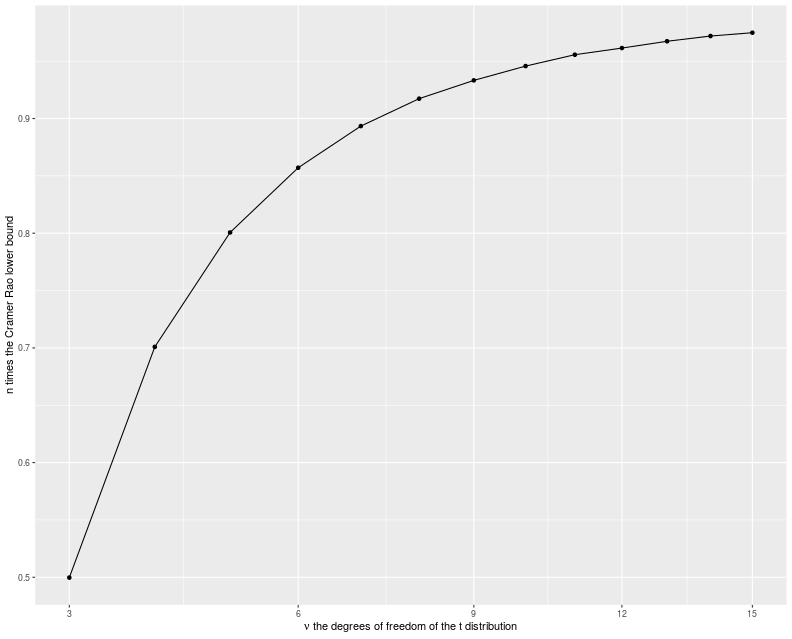## Trimmed mean

The other estimator we will try is the trimmed mean. First assume some $$\gamma$$ between 0 and $$1/2$$ is chosen. Then compute the $$\gamma$$ and $$1-\gamma$$ sample quantiles of the observed $$x_i$$. Take the mean of the subset of the $$x_i$$ which are between those two quantiles (there are approximately $$n\left(1 - 2\gamma\right)$$ such $$x_i$$). As $$\gamma \to 1/2$$ you should recover the sample median, while as $$\gamma \to 0$$ you get the (untrimmed) sample mean.

It turns out that the sample trimmed mean has a standard error of approximately

$$\frac{s_w}{\left(1-2\gamma\right)\sqrt{n}},$$

where $$s_w$$ is the square root of the Winsorized variance. (Approximately here means there is an extra term that is of the order $$1/n$$.) Winsorizing is like trimming, but you cap the values rather than throw them away. That is define $$\hat{x} = \max{\left(q_{\gamma},\min{\left(q_{1-\gamma},x\right)}\right)}$$, where $$q_{\alpha}$$ is the population $$\alpha$$ quantile of the distribution of $$x$$. Then the variance of $$\hat{x}$$ is the Winsorized variance of $$x$$.

For our toy problem, since we know the density of $$x$$ we can easily estimate the Winsorized variance, and thus we can find the $$\gamma$$ that gives the smallest standard error. (c.f. John Cook's post on the efficiency of the median versus the mean for $$t$$ variates.)

Here is some code to compute these things:

suppressMessages({
library(dplyr)
library(tidyr)
library(magrittr)
})
trim_x <- function(x,alpha=0.2,beta=1-alpha) {
stopifnot(alpha > 0, alpha < 0.5,beta > 0.5, beta < 1)
# trimmed mean
qs <- quantile(x,c(alpha,beta))
tx <- x[(x >= min(qs)) & (x <= max(qs))]
}
trim_mean <- function(x,alpha=0.2,beta=1-alpha) {
mean(trim_x(x,alpha=alpha,beta=beta))
}
# find the standard error of the gamma-trimmed mean on a t variable
# find the approximate standard error of the gamma-trimmed mean on a
# shifted, scaled $t$ distribution.
tse <- function(gamma,nu,ncut=9999) {
stopifnot(gamma > 0,gamma < 0.5)
sg <- sqrt(1 - (2/nu))
tlims <- qt(c(gamma,1-gamma),df=nu)
xs <- sg * seq(from=min(tlims),to=max(tlims),length.out=ncut)
delx <- diff(xs)
ys <- xs^2 * (1/sg) * dt(xs/sg,df=nu)
# compute the Winsorized variance:
winsv <- 0.5 * (sum(ys[-1] * delx) + sum(ys[-ncut] * delx)) + gamma * sum((sg*tlims)^2)
sqrt(winsv) / (1 - 2 * gamma)
}
# find the optimal trimming for a t_nu random variable
min_tes <- function(nu,eps=0.0001,ncut=9999) {
f <- function(x) tse(x,nu=nu,ncut=ncut)
ys <- optimize(f, interval=c(eps,0.5-eps))
ys$minimum }  Here is a plot of the approximately optimal $$\gamma$$ as a function of $$\nu$$: suppressMessages({ library(dplyr) library(tidyr) library(ggplot2) }) params <- data.frame(nu=unique(c(seq(4.01,4.09,by=0.01), seq(4.1,5.0,by=0.1), seq(5.25,10,by=0.25), seq(11,20,by=1), seq(22,50,by=2), seq(55,100,by=5)))) %>% group_by(nu) %>% summarize(gamma=min_tes(nu)) %>% ungroup() ph <- params %>% ggplot(aes(nu,gamma)) + geom_point() + stat_smooth(method='lm') + scale_x_sqrt() + scale_y_log10() + labs(x=expression(paste(nu,', the degrees of freedom of the t distribution')), y=expression(paste('the ',gamma,' which gives the lowest standard error for the trimmed mean'))) print(ph)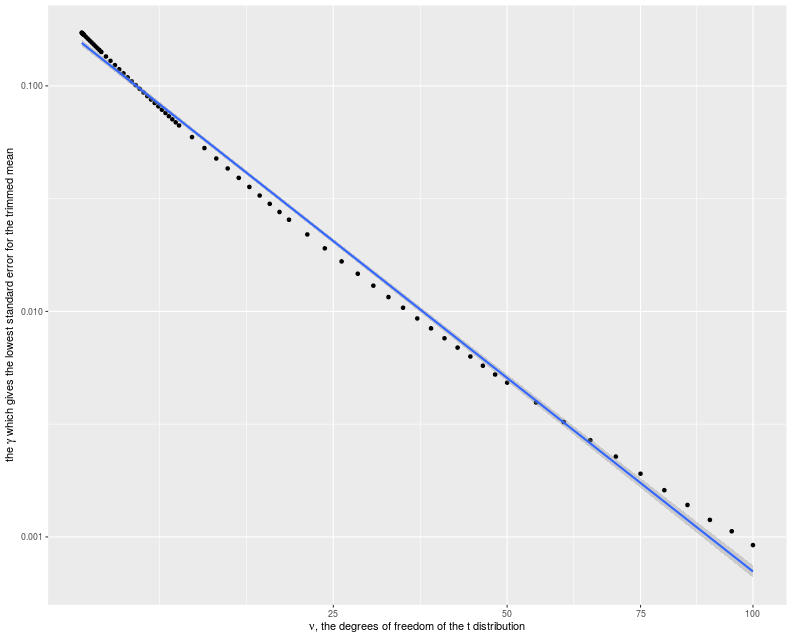We should like to know how efficient the trimmed mean is compared to the Cramér Rao bound. So here we assume a sample size of, say 252, and compute both approximate standard error of the optimally trimmed mean, and the Cramér Rao bound. We see that they are very close to each other. n <- 252 params <- data.frame(nu=unique(c(seq(4.01,4.09,by=0.01), seq(4.1,5.0,by=0.1), seq(5.25,10,by=0.25), seq(11,20,by=1), seq(22,50,by=2), seq(55,100,by=5)))) %>% group_by(nu) %>% summarize(gamma=min_tes(nu)) %>% ungroup() %>% group_by(nu,gamma) %>% # add on the estimated tse mutate(trim_se=tse(gamma,nu)) %>% ungroup() %>% # add on the crlb group_by(nu) %>% mutate(crbit=sqrt(crlb(nu=nu))) %>% ungroup() %>% mutate(trim_se = trim_se / sqrt(n), crlb = crbit / sqrt(n)) %>% dplyr::select(-crbit) ph <- params %>% tidyr::gather(key=series,value=value,trim_se,crlb) %>% mutate(series=case_when(.$series=='trim_se' ~ 'approximate standard error of trimmed mean',
.$series=='crlb' ~ 'Cramer Rao lower bound', TRUE ~ 'bad code')) %>% ggplot(aes(nu,value,color=series)) + geom_line() + scale_x_log10() + labs(x=expression(paste(nu,', the degrees of freedom of the t distribution')), y='estimator of standard error', title='comparison of trimmed mean standard error to the lower bound') print(ph)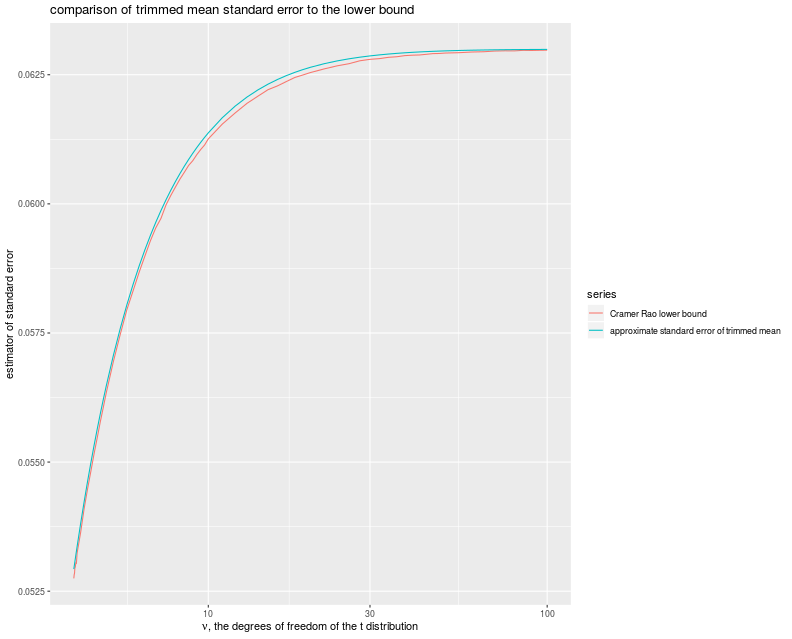## Simulations Let's throw all these things together into some simulations. We will draw variates from a $$t$$ distribution with $$\nu$$ degrees of freedom known, rescale to unit variance, then add on $$\mu$$. We will then compute the MLE of the mean, and the trimmed mean with near optimal trimming. To make things interesting, we will also compute the drawdown estimator of Signal Noise ratio, even though it is at a disadvantage, since it must estimate the volatility and the degrees of freedom. We will compute the mean and the Sharpe ratio for reference. We will also compute, as a function of $$\nu$$, the Cramér Rao bound and the approximate theoretical standard error of the trimmed mean, for reference: suppressMessages({ library(dplyr) library(tidyr) library(tibble) library(sharpeRratio) library(maxLik) library(moments) # https://cran.r-project.org/web/packages/doFuture/vignettes/doFuture.html library(doFuture) registerDoFuture() plan(multiprocess) }) # only works for scalar pzeta: onesim <- function(n,pzeta,nu,gamma,...) { x <- pzeta + sqrt(1 - (2/nu)) * rt(n,df=nu) mux <- mean(x) sdx <- sd(x) mome <- mux / sdx trym <- trim_mean(x,alpha=gamma) mlee <- simp_mle_est(x,nu=nu) ddsr <- unlist(lapply(pzeta-pzeta,function(addon) { sim <- sharpeRratio::estimateSNR(x+addon) sim$SNR
}))
cbind(pzeta,mux,mome,ddsr,mlee,trym)
}
repsim <- function(nrep,n,pzeta,nu,gamma) {
dummy <- invisible(capture.output(jumble <- replicate(nrep,onesim(n=n,pzeta=pzeta,nu=nu,gamma=gamma)),file='/dev/null'))
retv <- aperm(jumble,c(1,3,2))
dim(retv) <- c(nrep * length(pzeta),dim(jumble))
colnames(retv) <- colnames(jumble)
invisible(as.data.frame(retv))
}
manysim <- function(nrep,n,pzeta,nu,gamma,nnodes=5) {
if (nrep > 2*nnodes) {
# do in parallel.
nper <- table(1 + ((0:(nrep-1) %% nnodes)))
retv <- foreach(i=1:nnodes,.export = c('n','pzeta','nu','gamma','onesim','repsim')) %dopar% {
repsim(nrep=nper[i],n=n,pzeta=pzeta,nu=nu,gamma=gamma)
} %>%
bind_rows()
} else {
retv <- repsim(nrep=nrep,n=n,pzeta=pzeta,nu=nu,gamma=gamma)
}
retv
}

ope <- 252
pzetasq <- c(0,1/4,1,4) / ope
pzeta <- sqrt(pzetasq)

nus <- tibble::tribble(~nu,4.2,4.75,7,16)

# get bounds
bounds <- nus %>%
group_by(nu) %>%   # figure out optimal gamma
mutate(gamma=min_tes(nu,eps=0.0001)) %>%
ungroup() %>%
group_by(nu,gamma) %>%   # add on the estimated tse
mutate(trim_se=tse(gamma,nu)) %>%
ungroup() %>%   # add on the crlb
group_by(nu) %>%
mutate(crbit=sqrt(crlb(nu=nu))) %>%
ungroup()

params <- tidyr::crossing(tibble::tribble(~n,128,256,512,1024),
tibble::tibble(pzeta=pzeta),
nus) %>%
left_join(bounds,by='nu') %>%
mutate(trim_se = trim_se / sqrt(n)) %>%
mutate(crbound = crbit / sqrt(n)) %>%
dplyr::select(-crbit)

# run a bunch
nrep <- 500
set.seed(1234)
system.time({
results <- params %>%
group_by(n,pzeta,nu,gamma,trim_se,crbound) %>%
summarize(sims=list(manysim(nrep=nrep,nnodes=8,
pzeta=pzeta,n=n,nu=nu,gamma=gamma))) %>%
ungroup() %>%
tidyr::unnest() %>%
dplyr::select(-pzeta1)
})

   user  system elapsed
5560.71 2864.67 1247.19


Now I collect the results of the simulation, computing the bias, standard error and root mean square error of the two methods of computing Sharpe, and joining on with the population moment values.

# summarize the results
sumres <- results %>%
tidyr::gather(key=stat,value=value,-n,-nu,-gamma,-pzeta,-trim_se,-crbound) %>%
mutate(error=value - pzeta) %>%
group_by(n,nu,gamma,pzeta,stat,trim_se,crbound) %>%
summarize(mean=mean(value,na.rm=TRUE),
bias=mean(error,na.rm=TRUE),
sd=sd(value,na.rm=TRUE),
rmse=sqrt(mean(error^2,na.rm=TRUE))) %>%
ungroup() %>%
tidyr::gather(key=metric,value=value,mean,bias,sd,rmse) %>%
mutate(ref=mome) %>%
tidyr::gather(key=stat,value=value,mux,mome,ddsr,mlee,trym) %>%
mutate(ratio=value / ref) %>%
mutate(stat=case_when(.$stat=='mome' ~ 'moment based Sharpe', .$stat=='ddsr' ~ 'drawdown based SNR estimator',
.$stat=='mux' ~ 'vanilla mean', .$stat=='mlee' ~ 'MLE of mean',
.$stat=='trym' ~ 'optimal trim mean', TRUE ~ 'bad code')) %>% mutate(annualized SNR=signif(pzeta * sqrt(ope),digits=2)) %>% mutate(ex_kurt=6 / (nu - 4)) %>% rename(excess kurtosis=ex_kurt) %>% mutate(excess kurtosis=signif(excess kurtosis,digits=3))  Now I plot the ratio of the empirical RMSEs to the Cramér Rao lower bound versus the number of days of data, with facet columns for $$\nu$$ (equivalently, the excess kurtosis), and facet rows for the Signal to Noise ratio: ph <- sumres %>% filter(metric=='rmse') %>% dplyr::select(-ratio,-trim_se) %>% mutate(ratio=value / crbound) %>% mutate(annualized SNR=factor(annualized SNR)) %>% ggplot(aes(n,ratio,color=stat)) + geom_line() + geom_jitter(width=0,height=0.01,alpha=0.5) + scale_x_log10() + scale_y_sqrt() + geom_hline(yintercept=1,linetype=2,alpha=0.5) + facet_grid(annualized SNR~excess kurtosis+nu,labeller=label_both) + labs(y='ratio of empirical RMSE to the Cramer Rao lower bound', x='number of days of data', title='ratio of empirical RMSE to Cramer Rao lower bound, t returns') print(ph)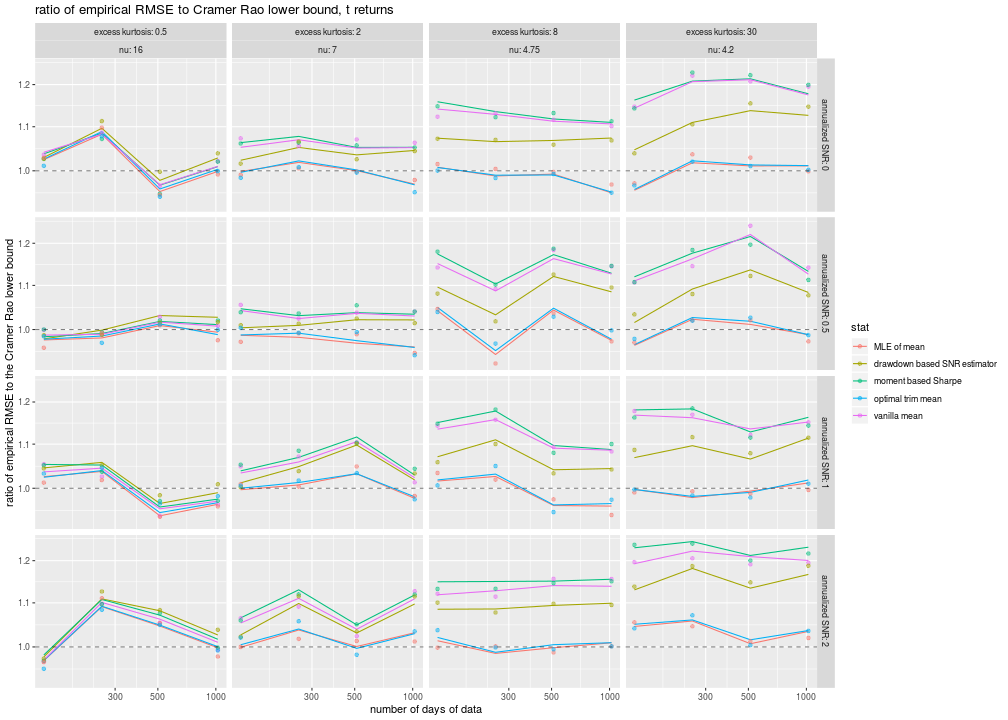A few conclusions from this plot, in increasing order of surprise: • The trimmed mean and the MLE of the mean have nearly indistinguishable RMSE. I have jittered the points slightly in the hope of making the points visible. • The trimmed mean outperforms the vanilla mean in terms of lower RMSE, with the more noticeable effect for more leptokurtic distributions on the right. The trimmed mean nearly achieves the lower bound (and is sometimes a little less, probably due to sampling variation). • The vanilla mean and the moment-based Sharpe have nearly the same RMSE. That is, the estimation of the volatility contributes very little to the total error. • The drawdown estimator, despite having two hands tied behind its back, achieves lower RMSE than the Cramér Rao lower bound. This 'super efficiency' is most visible for low Signal-Noise, high kurtosis situations, as in the upper right corner of our plots. For higher SNR, the bias of the drawdown estimator takes over, and the drawdown estimator underperforms even the sample mean. What? How does an estimator achieve a lower RMSE than the Cramér Rao lower bound? Especially one that is operating at a loss, estimating more than is necessary for the CRLB? Recall that one of the technical conditions of the Cramér Rao theory is that the distribution of errors of the estimator must be independent of the parameter being estimated. I call this the 'no stopped clocks' condition: just as a stopped clock is right twice a day, an estimator which is biased towards certain values can seem to be superefficient when tested near those values. I mentioned in a previous post that I suspect the drawdown estimator is employing a kind of 'unconscious shrinkage', as it has been trained on data which have Signal Noise ratio around -1 to 1 or so in annualized terms. The simulations here strongly suggest this kind of shrinkage. Practitioners should exercise extreme caution before using the drawdown estimator! ## Honest Sharpe simulations Above we performed simulations to estimate the mean of returns with known volatility and kurtosis. An 'honest' estimator does not know these in advance. As above, I create a trimmed mean Sharpe, which estimates the $$\nu$$ parameter by computing the excess kurtosis, then uses the optimal $$\gamma$$ for that $$\nu$$. As we expect little noise from the volatility estimate, we simply normalize by the vanilla sample standard deviation. We also implement an MLE estimator which assumes a shifted, scaled $$t$$ distribution, but estimates the $$\nu, \sigma$$ and $$\mu$$ separately. We test these, and the vanilla Sharpe ratio and the drawdown estimator, on $$t$$ returns, but also on skewed returns from a Lambert W x Gaussian distribution. Here are the simulations: suppressMessages({ library(dplyr) library(tidyr) library(tibble) library(sharpeRratio) library(maxLik) library(moments) # https://cran.r-project.org/web/packages/doFuture/vignettes/doFuture.html library(doFuture) registerDoFuture() plan(multiprocess) }) # log likelihood of # (x - mu) / sg ~ t(df=nu) tlik <- function(param,x) { mu <- param sg <- param nu <- max(4,param) t <- (x-mu) / sg ## also equal to: #n <- length(x) #retv <- n * (lgamma((nu+1)/2) - lgamma(nu/2)- 0.5 * log(nu * pi) - log(sg)) - ((nu+1)/2) * sum(log(1 + (t^2)/nu)) sum(dt(t,df=nu,log=TRUE) - log(sg)) } # gradient of same tlikgrad <- function(param,x) { mu <- param sg <- param nu <- max(4,param) t <- (x-mu) / sg n <- length(x) gradmu <- ((nu+1)/2) * sum(2 * t / (1 + (t^2)/nu)) gradsg <- - (n / sg) + ((nu+1)/2) * sum(2 * t / ((sg^2) * (1 + (t^2)/nu))) gradnu <- (n/2) * (digamma((nu+1)/2) - digamma(nu/2) - (1/nu)) - (1/2) * sum(log(1 + (t^2)/nu)) + ((nu+1)/2) * sum(((t^2)/(n^2)) / (1 + (t^2)/nu)) c(gradmu,gradsg,gradnu) } mle_est <- function(x) { exk <- moments::kurtosis(x) - 3 nuest <- 4 + 6/max(0.0001,exk) param0 <- c(mean(x),sd(x),nuest) A <- matrix(c(0,1,0,0,0,1),nrow=2,byrow=TRUE) B <- matrix(c(0,-4),nrow=2) cons <- list(ineqA = A,ineqB = B) foo <- maxLik(logLik=tlik,grad=tlikgrad,x=x,start=param0,constraints=cons,method='BFGS') # now compute 'sharpe' param <- foo$estimate
truesg <- param * sqrt(param / (param-2))
param / truesg
}
trim_x <- function(x,alpha=0.2,beta=1-alpha) {
stopifnot(alpha > 0, alpha < 0.5,beta > 0.5, beta < 1)
# trimmed mean
qs <- quantile(x,c(alpha,beta))
tx <- x[(x >= min(qs)) & (x <= max(qs))]
}
trim_mean <- function(x,alpha=0.2,beta=1-alpha) {
mean(trim_x(x,alpha=alpha,beta=beta))
}
trim_sharpe <- function(x,alpha=0.2,beta=1-alpha) {
# trimmed mean
tmu <- trim_mean(x,alpha=alpha,beta=beta)
# 2FIX: also compute standard errors?
retv <- tmu / sd(x)
}
# find the standard error of the gamma-trimmed mean on a t variable
# find the approximate standard error of the gamma-trimmed mean on a
# shifted, scaled $t$ distribution.
tse <- function(gamma,nu,ncut=9999) {
stopifnot(gamma > 0,gamma < 0.5)
sg <- sqrt(1 - (2/nu))
tlims <- qt(c(gamma,1-gamma),df=nu)
xs <- sg * seq(from=min(tlims),to=max(tlims),length.out=ncut)
delx <- diff(xs)
ys <- xs^2 * (1/sg) * dt(xs/sg,df=nu)
# compute the Winsorized variance:
winsv <- 0.5 * (sum(ys[-1] * delx) + sum(ys[-ncut] * delx)) + gamma * sum((sg*tlims)^2)
sqrt(winsv) / (1 - 2 * gamma)
}
# find the optimal trimming for a t_nu random variable
min_tes <- function(nu,eps=0.0001,ncut=9999) {
f <- function(x) tse(x,nu=nu,ncut=ncut)
ys <- optimize(f, interval=c(eps,0.5-eps))
ys$minimum } opt_gammas <- data.frame(nu=unique(c(seq(4.01,4.09,by=0.005), seq(4.1,5.0,by=0.05), seq(5.25,10,by=0.25), seq(11,20,by=1), seq(22,50,by=2), seq(55,100,by=5)))) %>% group_by(nu) %>% summarize(gamma=min_tes(nu)) %>% ungroup() %>% mutate(ex_kurt=6 / (nu - 4)) opt_trim_sharpe <- function(x) { exk <- moments::kurtosis(x) - 3 if (exk <= min(opt_gammas$ex_kurt)) {
# vanilla sharpe
retv <- mean(x) / sd(x)
} else {
if (exk <= max(opt_gammas$ex_kurt)) { best <- approx(x=opt_gammas$ex_kurt,y=opt_gammas$gamma,xout=exk) gam <- best$y
} else {
gam <- max(opt_gammas$gamma) } retv <- trim_sharpe(x,alpha=gam) } retv } onesim <- function(n,pzeta,gen=rnorm,...) { # works on one pzeta x <- gen(n) + pzeta mome <- mean(x) / sd(x) trym <- opt_trim_sharpe(x) mlee <- mle_est(x) ddsr <- sharpeRratio::estimateSNR(x)$SNR
cbind(pzeta,mome,ddsr,mlee,trym)
}

repsim <- function(nrep,n,pzeta,gen=rnorm) {
dummy <- invisible(capture.output(jumble <- replicate(nrep,onesim(n=n,pzeta=pzeta,gen=gen)),file='/dev/null'))
retv <- aperm(jumble,c(1,3,2))
dim(retv) <- c(nrep * length(pzeta),dim(jumble))
colnames(retv) <- colnames(jumble)
invisible(as.data.frame(retv))
}
manysim <- function(nrep,n,pzeta,gen=rnorm,nnodes=5) {
if (nrep > 2*nnodes) {
# do in parallel.
nper <- table(1 + ((0:(nrep-1) %% nnodes)))
retv <- foreach(i=1:nnodes,.export = c('n','pzeta','gen','onesim','repsim')) %dopar% {
repsim(nrep=nper[i],n=n,pzeta=pzeta,gen=gen)
} %>%
bind_rows()
} else {
retv <- repsim(nrep=nrep,n=n,pzeta=pzeta,gen=gen)
}
retv
}
tgen <- function(df) {
rescal <- sqrt((df - 2)/df)
function(n) { rescal * rt(n,df=df) }
}
#Lambert x Gaussian
gen_lambert_w <- function(n,dl = 0.1,mu = 0,sg = 1) {
require(LambertW,quietly=TRUE)
force(dl)
force(mu)
force(sg)
Gauss_input = create_LambertW_input("normal", beta=c(0,1))
params = list(delta = c(0), gamma=c(dl), alpha = 1)
LW.Gauss = create_LambertW_output(Gauss_input, theta = params)
#get the moments of this distribution
moms <- mLambertW(beta=c(0,1),distname=c("normal"),delta = 0,gamma = dl, alpha = 1)
if (!is.null(LW.Gauss$r)) { # API changed in 0.5: samp <- LW.Gauss$r(n=n)
} else {
samp <- LW.Gauss$rY(params)(n=n) } samp <- mu + (sg/moms$sd) * (samp - moms$mean) } lamgen <- function(delta) { lapply(delta,function(del) { function(n) gen_lambert_w(n=n,dl=del,mu=0,sg=1) }) } moms_lambert_w <- function(dl = 0.1,mu = 0,sg = 1) { require(LambertW,quietly=TRUE) Gauss_input = create_LambertW_input("normal", beta=c(0,1)) params = list(delta = c(0), gamma=c(dl), alpha = 1) LW.Gauss = create_LambertW_output(Gauss_input, theta = params) #get the moments of this distribution moms <- mLambertW(beta=c(0,1),distname=c("normal"),delta = 0,gamma = dl, alpha = 1) moms$mean <- mu
moms$sd <- sg return(as.data.frame(moms)) } generators <- tibble::tribble(~name, ~gen, ~skewness, ~ex_kurt, 'gaussian', rnorm, 0, 0, 't_6', tgen(6), 0, 6 / (6-4), 't_4.75', tgen(4.75), 0, 6 / (4.75-4), 't_4.1', tgen(4.1), 0, 6 / (4.1-4)) %>% rbind( tibble::tribble(~delta,0.012,0.25,0.5) %>% group_by(delta) %>% summarize(moms=list(moms_lambert_w(dl=delta))) %>% ungroup() %>% mutate(name=paste0('lambert_',delta)) %>% unnest(moms) %>% mutate(gen=(lamgen(delta))) %>% mutate(ex_kurt=kurtosis-3) %>% dplyr::select(-mean,-sd,-kurtosis,-delta) ) ope <- 252 pzetasq <- c(0,1/4,1,4) / ope pzeta <- sqrt(pzetasq) params <- tidyr::crossing(tibble::tribble(~n,128,256,512,1024), tibble::tibble(pzeta=pzeta), generators) # run a bunch nrep <- 250 set.seed(1234) system.time({ results <- params %>% group_by(n,pzeta,name,skewness,ex_kurt) %>% summarize(sims=list(manysim(nrep=nrep,nnodes=8, pzeta=pzeta,n=n,gen=gen[]))) %>% ungroup() %>% tidyr::unnest() %>% dplyr::select(-pzeta1) })   user system elapsed 9732.12 2510.39 1867.67  Now I collect the results of the simulation, computing the bias, standard error and root mean square error of the two methods of computing Sharpe, and joining on with the population moment values. # summarize the results. sumres <- results %>% tidyr::gather(key=stat,value=value,-n,-name,-skewness,-ex_kurt,-pzeta) %>% mutate(error=value - pzeta) %>% group_by(n,name,skewness,ex_kurt,pzeta,stat) %>% summarize(mean=mean(value,na.rm=TRUE), bias=mean(error,na.rm=TRUE), sd=sd(value,na.rm=TRUE), rmse=sqrt(mean(error^2,na.rm=TRUE))) %>% ungroup() %>% tidyr::gather(key=metric,value=value,mean,bias,sd,rmse) %>% tidyr::spread(key=stat,value=value) %>% mutate(ref=mome) %>% tidyr::gather(key=stat,value=value,mome,ddsr,mlee,trym) %>% mutate(ratio=value / ref) %>% mutate(stat=case_when(.$stat=='mome' ~ 'moment based estimator',
.$stat=='ddsr' ~ 'drawdown based estimator', .$stat=='mlee' ~ 'MLE based estimator',
.$stat=='trym' ~ 'optimal trim mean estimator', TRUE ~ 'bad code')) %>% mutate(annualized SNR=signif(pzeta * sqrt(ope),digits=2)) %>% mutate(skewness=signif(skewness,digits=3)) %>% mutate(asymmetric=!grepl('^(gauss|t_\\d)',name)) %>% rename(excess kurtosis=ex_kurt) %>% mutate(excess kurtosis=signif(excess kurtosis,digits=3))  Now I plot the ratio of the empirical RMSE to that of the moment-based estimator, and plot versus the number of days of data, with facet rows for the annualized Signal Noise ratio and columns for the skew and excess kurtosis of the distribution. I then also plot the bias of all four estimators # plot rmses ph <- sumres %>% filter(metric=='rmse') %>% filter(!grepl('moment based',stat)) %>% mutate(annualized SNR=factor(annualized SNR)) %>% ggplot(aes(n,ratio,color=stat)) + geom_line() + geom_point() + scale_x_log10() + scale_y_log10() + geom_hline(yintercept=1,linetype=2,alpha=0.5) + facet_grid(annualized SNR~asymmetric+skewness+excess kurtosis,labeller=label_both) + labs(y='ratio of empirical RMSE to that of moment estimator', x='number of days of data', title='ratio of empirical RMSE ratio estimators of Signal Noise ratio, various returns distributions') print(ph)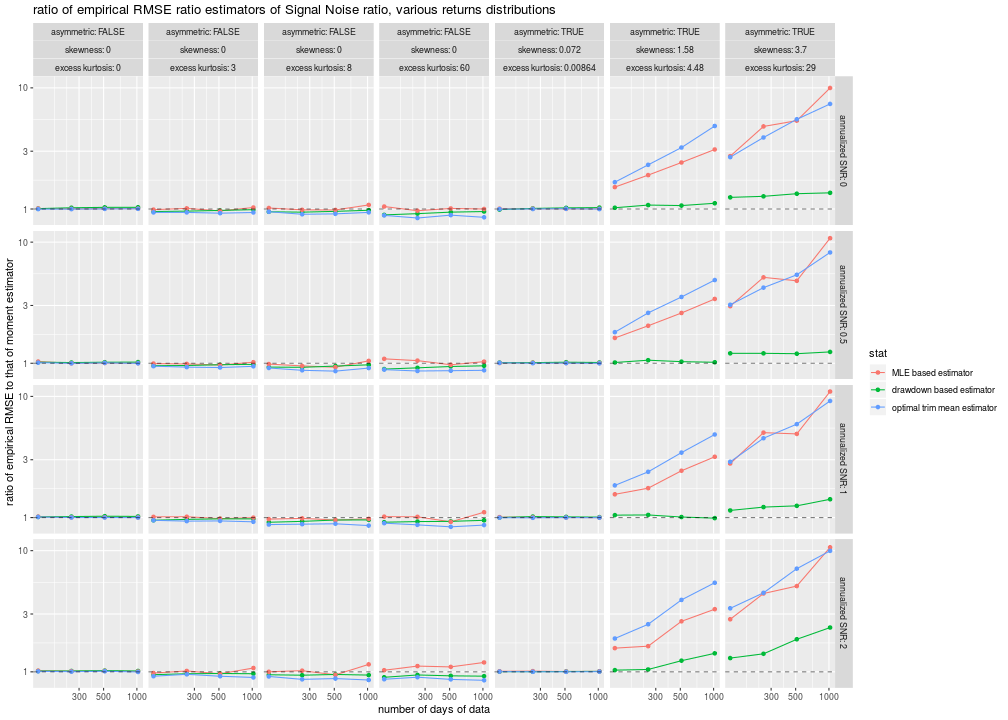# plot bias ph <- sumres %>% filter(metric=='bias') %>% mutate(annualized SNR=factor(annualized SNR)) %>% ggplot(aes(n,value,color=stat)) + geom_line() + geom_point() + scale_x_log10() + geom_hline(yintercept=0,linetype=2,alpha=0.5) + facet_grid(annualized SNR~skewness+excess kurtosis,labeller=label_both) + labs(y='empirical bias', x='number of days of data', title='empirical bias of estimators of Signal Noise ratio, various returns distribugtions') print(ph)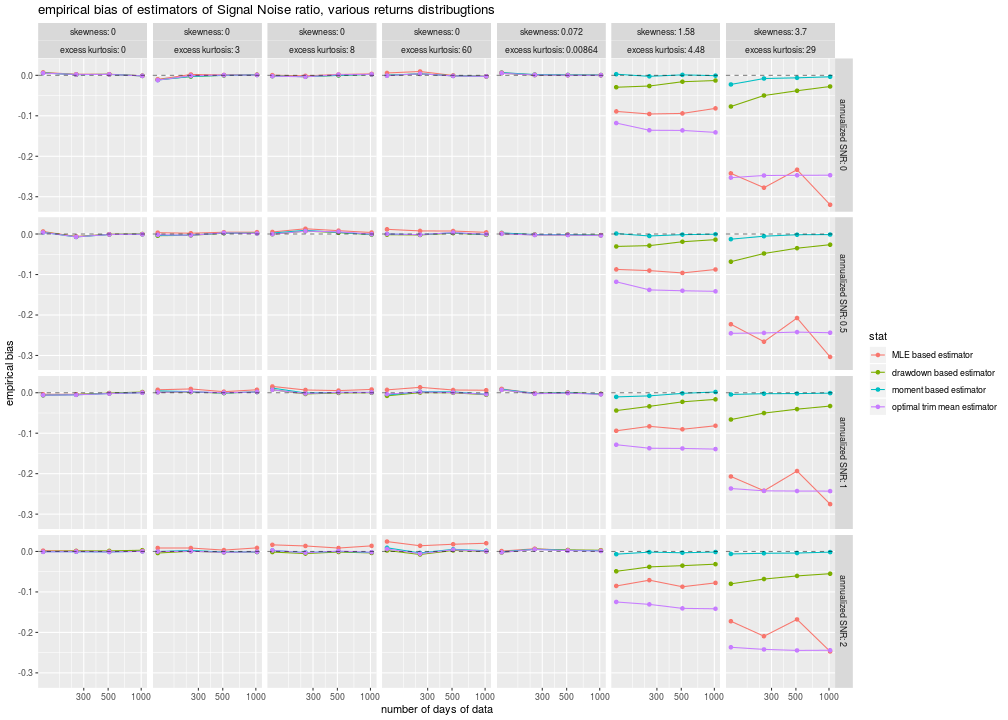Some observations: • For the symmetric distributions, the trimmed Sharpe outperforms the MLE, which only does about as well as the vanilla Sharpe. Neither of them gives the performance of the dradown estimator, except for the high SNR case, perhaps due to shrinkage. • Both the trimmed Sharpe and the MLE estimators are awful for skewed distributions, much worse than the drawdown estimator, and are horribly biased. They simply should not be used. The question remains: can we improve on the vanilla Sharpe to deal with higher order moments, while avoiding bias, keeping some theoretical guarantees, and not shrinking to zero? Click to read and post comments #### Feb 24, 2018 ## A Sharper Sharpe II : Skewed Distributions Previously we performed some simulations to test Damien Challet's 'Sharper estimator' of the Signal-Noise Ratio, which we define as the ratio of population values $$\mu/\sigma$$. In that study, we drew returns from the (central) $$t$$ distribution, as Challet did in his study, and in the calibration of his R package, sharpeRratio. Our simulations indicated that this new 'drawdown estimator' seemed to have lower standard error (and root mean square error) than the traditional moment-based estimator for leptokurtotic returns. This is not to say there were no wrinkles. For large effect sizes (signal noise times square root number of observations), the drawdown estimator had much worse RMSE than the moment estimator, which I conjectured might be due to 'being out of range' of the original simulations used by the software's author to calibrate the spline functions used in sharpeRratio. However, the drawdown estimator has a few known deficiencies. For one, it is not well understood theoretically: we have no solid understanding why it should have lower standard error than the moment estimator; nor do we know if it should be unbiased; and we do not understand how it might react in the presence of skewed returns. This last question, at least, we can explore via simulations. Here we draw returns from Lambert W x Gaussian distribution with a different $$\delta$$ parameter to achieve different values of skew and kurtosis. Here we run the simulations, letting the number of days of data run from 128 to 1024, letting the annualized Signal Noise ratio run from 0 to 2, and sweeping the $$\delta$$ parameter from 0 (which is the Gaussian case) to 0.5, which causes the population skewness to run from 0 to around 3.7, and the population excess kurtosis to vary up to about 30. (For reference the daily returns of the S&P 500 has skewness around -1, and excess kurtosis around 25.) For each setting, we run 500 simulations. Here is the code: suppressMessages({ library(dplyr) library(tidyr) library(tibble) library(sharpeRratio) library(LambertW) # https://cran.r-project.org/web/packages/doFuture/vignettes/doFuture.html library(doFuture) registerDoFuture() plan(multiprocess) }) #Lambert x Gaussian gen_lambert_w <- function(n,dl = 0.1,mu = 0,sg = 1) { require(LambertW,quietly=TRUE) Gauss_input = create_LambertW_input("normal", beta=c(0,1)) params = list(delta = c(0), gamma=c(dl), alpha = 1) LW.Gauss = create_LambertW_output(Gauss_input, theta = params) #get the moments of this distribution moms <- mLambertW(beta=c(0,1),distname=c("normal"),delta = 0,gamma = dl, alpha = 1) if (!is.null(LW.Gauss$r)) {
# API changed in 0.5:
samp <- LW.Gauss$r(n=n) } else { samp <- LW.Gauss$rY(params)(n=n)
}
samp <- mu  + (sg/moms$sd) * (samp - moms$mean)
}
moms_lambert_w <- function(dl = 0.1,mu = 0,sg = 1) {
require(LambertW,quietly=TRUE)
Gauss_input = create_LambertW_input("normal", beta=c(0,1))
params = list(delta = c(0), gamma=c(dl), alpha = 1)
LW.Gauss = create_LambertW_output(Gauss_input, theta = params)
#get the moments of this distribution
moms <- mLambertW(beta=c(0,1),distname=c("normal"),delta = 0,gamma = dl, alpha = 1)
moms$mean <- mu moms$sd <- sg
return(as.data.frame(moms))
}

onesim <- function(n,pzeta,gen=rnorm,...) {
x <- gen(n) + pzeta
mux <- mean(x)
sdx <- sd(x)
mome <- (mux + (pzeta - pzeta)) / sdx
sim$SNR })) cbind(pzeta,mome,ddsr) } repsim <- function(nrep,n,pzeta,gen=rnorm) { dummy <- invisible(capture.output(jumble <- replicate(nrep,onesim(n=n,pzeta=pzeta,gen=gen)),file='/dev/null')) retv <- aperm(jumble,c(1,3,2)) dim(retv) <- c(nrep * length(pzeta),dim(jumble)) colnames(retv) <- colnames(jumble) invisible(as.data.frame(retv)) } manysim <- function(nrep,n,pzeta,delta=0,nnodes=5) { # will add the mean later gen <- function(n) gen_lambert_w(n,dl=delta,mu=0,sg=1) if (nrep > 2*nnodes) { # do in parallel. nper <- table(1 + ((0:(nrep-1) %% nnodes))) retv <- foreach(i=1:nnodes,.export = c('n','pzeta','gen','onesim','repsim')) %dopar% { repsim(nrep=nper[i],n=n,pzeta=pzeta,gen=gen) } %>% bind_rows() } else { retv <- repsim(nrep=nrep,n=n,pzeta=pzeta,gen=gen) } retv } ope <- 252 pzetasq <- c(0,1/4,1,4) / ope pzeta <- sqrt(pzetasq) params <- tidyr::crossing(tibble::tribble(~n,128,256,512,1024), tibble::tribble(~della,0,0.06,0.12,0.25,0.5), tibble::tibble(pzeta=pzeta)) # 13 takes 103 seconds on 6 nodes. nrep <- 500 set.seed(1234) system.time({ results <- params %>% group_by(n,della,pzeta) %>% summarize(sims=list(manysim(nrep=nrep,nnodes=4,pzeta=pzeta,n=n,delta=della))) %>% ungroup() %>% tidyr::unnest() })   user system elapsed 12299.99 6474.06 3154.82  Now I collect the results of the simulation, computing the bias, standard error and root mean square error of the two methods of computing Sharpe, and joining on with the population moment values. # compute the higher order moments moms <- results %>% distinct(della,pzeta) %>% group_by(della,pzeta) %>% summarize(moments=list(moms_lambert_w(dl=della,mu=pzeta,sg=1))) %>% ungroup() %>% unnest() %>% mutate(excess kurtosis=kurtosis - 3) # summarize the results sumres <- results %>% dplyr::select(-pzeta1) %>% tidyr::gather(key=stat,value=value,-n,-della,-pzeta) %>% mutate(error=value - pzeta) %>% group_by(n,della,pzeta,stat) %>% summarize(mean=mean(value,na.rm=TRUE), bias=mean(error,na.rm=TRUE), sd=sd(value,na.rm=TRUE), rmse=sqrt(mean(error^2,na.rm=TRUE))) %>% ungroup() %>% tidyr::gather(key=metric,value=value,mean,bias,sd,rmse) %>% left_join(moms,by=c('pzeta','della')) %>% tidyr::spread(key=stat,value=value) %>% mutate(ratio=ddsr/mome) %>% tidyr::gather(key=stat,value=value,ddsr,mome,ratio) %>% mutate(stat=case_when(.$stat=='mome' ~ 'moment based estimator',
.$stat=='ddsr' ~ 'drawdown based estimator', .$stat=='ratio' ~ 'ratio drawdown to moment estimators',
mutate(annualized SNR=signif(pzeta * sqrt(ope),digits=2)) %>%
mutate(skewness=signif(skewness,digits=3)) %>%
mutate(excess kurtosis=signif(excess kurtosis,digits=3))


I also computed the ratio of the RMSE values of the drawdown estimator to the moment estimator, calling this the 'inefficiency' of the drawdown estimator. When it is less than 1, the new drawdown estimator has smaller RMSE than the moment estimator. Here is a plot of those inefficiencies versus the number of days of data, with the SNR plotted by color, and column facets showing the population skewness and excess kurtosis. The far left column is essentially Gaussian returns.

# plot em
library(ggplot2)
ph <- sumres %>%
filter(metric=='rmse') %>%
filter(grepl('ratio',stat)) %>%
mutate(annualized SNR=factor(annualized SNR)) %>%
ggplot(aes(n,value,color=annualized SNR,group=annualized SNR)) +
geom_line() + geom_point() +
scale_x_log10() + scale_y_log10() +
geom_hline(yintercept=1,linetype=2,alpha=0.5) +
facet_grid(.~skewness+excess kurtosis,labeller=label_both) +
labs(y='"inefficiency" of drawdown estimator\n(ratio of drawdown RMSE to moment RMSE)',
x='number of days of data',
title='empirical RMSE ratio estimators of Signal Noise ratio, Lambda W x Gaussian returns')
print(ph)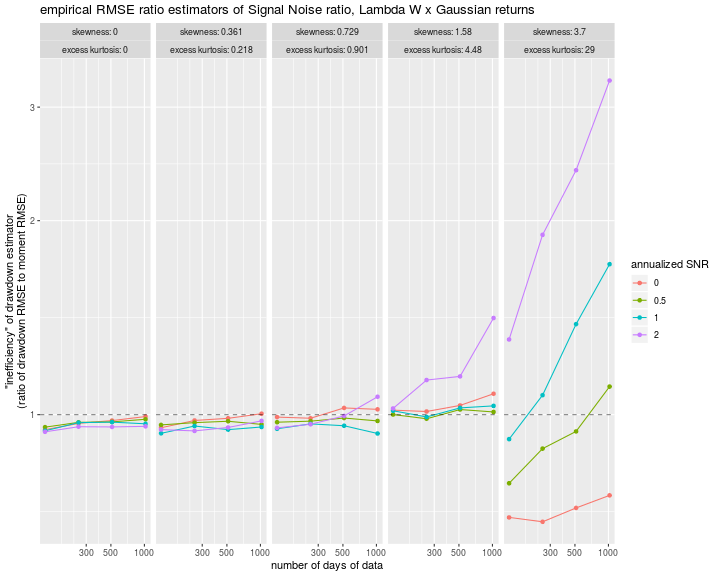It is hard to draw general conclusions from this plot other than that the drawdown estimator is much worse than the moment estimator for the high skew case when the SNR is large, but quite a bit better when the SNR is zero. What can that mean? Here we plot the bias of the two esimators: the mean value of the estimator minus the true SNR. We see that the moment estimator appears slightly biased for small sample sizes (A phenomenom that is well understood), but the drawdown estimator is truly awful for the case of high skew. When the SNR is around 2, it yields a value of perhaps 0.5, and the problem is not much improved by larger sample sizes. This does not instill a lot of confidence in the drawdown estimator!

# plot em
library(ggplot2)
ph <- sumres %>%
filter(metric=='bias') %>%
filter(!grepl('ratio',stat)) %>%
mutate(value=sqrt(ope) * value) %>% # annualized
ggplot(aes(n,value,color=stat)) +
geom_line() + geom_point() +
scale_x_log10() +
geom_hline(yintercept=0,linetype=2,alpha=0.5) +
facet_grid(annualized SNR~skewness+excess kurtosis,labeller=label_both) +
labs(y='bias of estimator of SNR, annualized',
x='number of days of data',
title='empirical bias of estimators of Signal Noise ratio, Lambda W x Gaussian returns')
print(ph)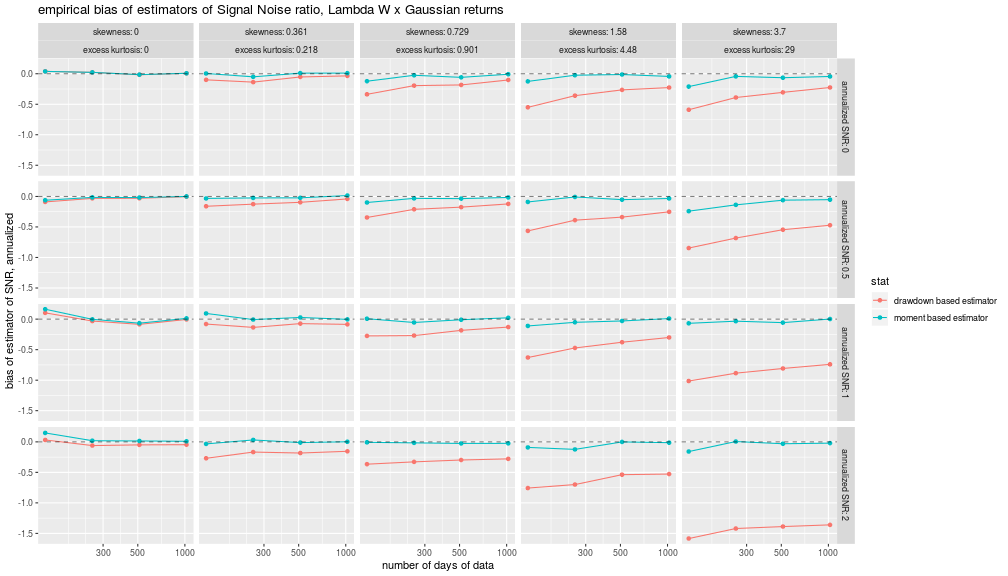Note that this problem appears to be inherent in the drawdown estimator itself: it works by rearranging the returns, then counting the number of times a new maximum and a new minimum of the resultant price series is seen, then repeats to get an estimate of the number of 'record highs' and 'record lows', which it then translates back into a SNR estimate. If the mean return is captured in a few days of outsize returns, as is the case for a skewed distribution, then the number of 'record highs' will be lower than seen in a symmetric distribution (the $$t$$ distribution used when calibrating the software). There will be bias.

On reflection, I suspect that the apparent improved performance for the drawdown estimator under symmetric returns, as seen previously, could be due to a kind of 'unconscious shrinkage' achieved by the design of the software. Suppose that the simulations which were used to calibrate the software did not include extremely large effect sizes (again, the SNR times square root days). As a whole, the software is 'expecting' smaller SNRs. When tested on an SNR of zero, it might achieve smaller standard error for this very reason. While this is an uncharitable take, perhaps, it still stands as fact that we know very little theoretically about the drawdown estimator, and using it without that theoretical understanding could be dangerous.